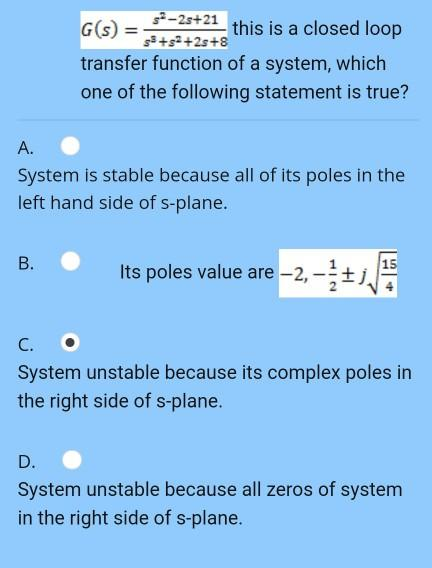# Question = 52-2s+21 G(s) this is a closed loop 33++2s+8 transfer function of a system, which one of the following statement is true? A. System is stable because all of its poles in the left hand side of s-plane. B. 15 Its poles value are -2, - C. System unstable because its complex poles in the right side of s-plane. D. System unstable because all zeros of system in the right side of s-plane.NJEOU5 The Asker · Electrical EngineeringTranscribed Image Text: = 52-2s+21 G(s) this is a closed loop 33++2s+8 transfer function of a system, which one of the following statement is true? A. System is stable because all of its poles in the left hand side of s-plane. B. 15 Its poles value are -2, - C. System unstable because its complex poles in the right side of s-plane. D. System unstable because all zeros of system in the right side of s-plane.
More
Transcribed Image Text: = 52-2s+21 G(s) this is a closed loop 33++2s+8 transfer function of a system, which one of the following statement is true? A. System is stable because all of its poles in the left hand side of s-plane. B. 15 Its poles value are -2, - C. System unstable because its complex poles in the right side of s-plane. D. System unstable because all zeros of system in the right side of s-plane.